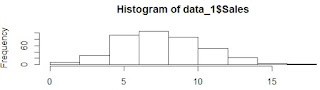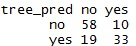## Pages

###Decision Science

We earlier covered the decision tree in R using {rpart} package in one of our previous articles. In R there are many packages that can be used for making a decision tree, out of which {tree} and {party} are my hot favorites. I will cover both of the packages one by one @ Ask Analytics.

Let's first learn usage of {tree} !

### Related articles:

Decision Tree in R- A Telecom Case Study

For the demonstration of decision tree with {tree} package, we would use a data Carseats which is inbuilt in the package ISLR. Let's first get the data.

# As usual, we first clean the environment
rm(list = ls())
# install the package if not already done
if (!require(ISLR)) install.packages("ISLR")
library(ISLR)
# let's make a copy of Carseats data into data_1
data_1 = Carseats

# Now let's install the package we need to focus in this article
if (!require(tree)) install.packages("tree")
library(tree)

# Let's first feel the data

It is a simulated data having sales of child car seats at 400 different stores.
Variables description
Sales : Unit sales (in thousands) at each location
CompPrice : Price charged by competitor at each location
Income : Community income level (in thousands of dollars)
Advertising : Local advertising budget for company at each location (in thousands of dollars)
Population : Population size in region (in thousands)
Price : Price company charges for car seats at each site
ShelveLoc : A factor with levels Bad, Good and Medium indicating the quality of the shelving location for the car seats at each site
Age : Average age of the local population
Education : Education level at each location
Urban : A factor with levels No and Yes to indicate whether the store is in an urban or rural location
US : A factor with levels No and Yes to indicate whether the store is in the US or not

Here the objective of decision tree will be to understand the sales pattern over rest of the variables and find out which are the variables that drive the sales.

For the purpose of making a classification tree, we first need to covert the Sales variable into a binary variable. Let's see how the sales in distributed :hist(data_1\$Sales)

We can see that the Sales is normally distributed and its value lies between 0 to 16 and mode is around 8. Let's bifurcate the variable in following fashion:

# We make a variable "high" which is "yes" when sale is more than or equal to 8, "no" otherwise
data_1\$high = as.factor(ifelse(data_1\$Sales>=8,"yes","no"))
# Also be drop the original Sales variable
data_1\$Sales = NULL

# Let's look at the data again

As a standard matter of modeling practices, we break the data into two parts :  Training data and Testing Data

• Training data : The datasets on which we train/build our model
• Testing data :  It is also called validation data where we check how good our model is performing
Also there is a third data for which actual value of predicted (Y) variable is not known and we are interested in predicting this value. This is the data for which this model is being built. But for learning purpose, we focus on the these two datasets.

Here we are breaking data into 70:30 ratio randomly.

# It is important to set a seed, if you don't define a seed every time you run the program, a different set of random numbers would be generated ... so seed kinda fixes the randomization

set.seed(222)
train = sample(1:nrow(data_1), nrow(data_1)*0.7)
Training_data =  data_1[train,]
Testing_data =  data_1[-train,]
rm(data_1,train)

# So now we would train our model on the Training_data

Tree_model = tree(high~. , Training_data)
plot(Tree_model)
text(Tree_model, pretty = 0)

# We got the tree, but the tree is too complex and not much legible. A such it is not a problem, as it is not required to read the tree for prediction point of view.

# We apply the model on the testing datasets and check how good it is doing

tree_pred = predict(Tree_model, Testing_data, type = "class")
mean(tree_pred != Testing_data\$high)

# and we get 0.3, which means that error rate is 30% i.e. in 30% of the observations actual vs. predicted values for "high" are not matching

Like we prune the trees and plants in our garden, similarly the decision trees are also pruned to get the most optimal result, which means maximum separation of "1" and "0"s with minimu,m branching.

set.seed(9)

cv_tree = cv.tree(Tree_model, FUN = prune.misclass)
plot(cv_tree\$size, cv_tree\$dev, type = 'b')

# Looking at the plot, we decide the optimla number of nodes required. We can try many values too.

# In this case, let's take 6 to be best one
pruned_tree = prune.misclass(Tree_model, best = 6)
plot(pruned_tree)

text(pruned_tree, pretty = 0)

# Now most important thing is: How to read this tree. It is very different type of but not that complicated to read.

For better understanding, take Training data into Excel, make pivot tables and check the numbers.

It says that if ShelvelLoc value are Bad or Medium, let's grow the tree otherwise it is highly likely that value of "high", which is target variable, is Yes

Then it encountered "Price" as next variable. If the Price is more than 127, in most cases "high" is no, else we need to grow the tree .... and so on.

Finally rules are made in the back end for prediction purpose.

# Now let's use the pruned tree for prediction

tree_pred = predict(pruned_tree, Testing_data, type = "class")
mean(tree_pred != Testing_data\$high)

# and we get the mis-classification rate as 24%, which can also be visualized astable(tree_pred,Testing_data\$high)

Where X axis is predicted and Y axis is observed.

Mis-classification rate = (10+19)/(58+33+10+19) = 24%
Hope the concept is clear to you.

Enjoy reading our other articles and stay tuned with us.

Kindly do provide your feedback in the 'Comments' Section and share as much as possible.

1.2.